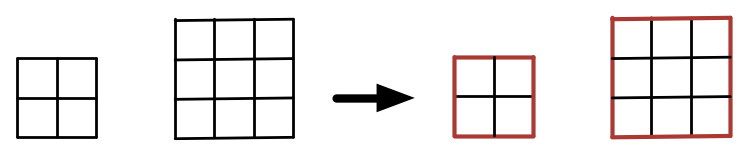# Red Perimeter 1 – Extend the Pattern

Probability Level 1Given $n \times n$ squares made out of $1 \times 1$ unit squares with the perimeter of the $n \times n$ square painted red, some of the $1 \times 1$ squares will be painted on 2 sides, some on only 1 side, and some won't be painted at all.

How many unit squares will be painted red on only 1 side in a $4 \times 4$ square (not pictured)?

This problem is part of Arron's set The Red Perimeter.

×